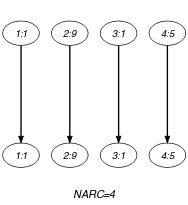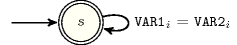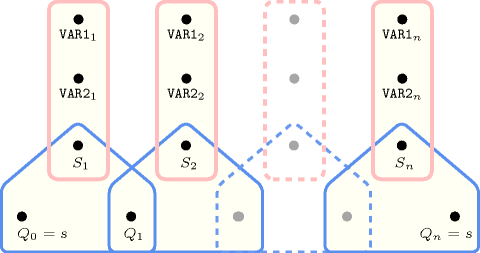## 5.228. lex_equal

Origin

Initially introduced for defining $\mathrm{𝚗𝚟𝚎𝚌𝚝𝚘𝚛}$

Constraint

$\mathrm{𝚕𝚎𝚡}_\mathrm{𝚎𝚚𝚞𝚊𝚕}\left(\mathrm{𝚅𝙴𝙲𝚃𝙾𝚁}\mathtt{1},\mathrm{𝚅𝙴𝙲𝚃𝙾𝚁}\mathtt{2}\right)$

Synonyms

$\mathrm{𝚎𝚚𝚞𝚊𝚕}$, $\mathrm{𝚎𝚚}$.

Arguments
 $\mathrm{𝚅𝙴𝙲𝚃𝙾𝚁}\mathtt{1}$ $\mathrm{𝚌𝚘𝚕𝚕𝚎𝚌𝚝𝚒𝚘𝚗}\left(\mathrm{𝚟𝚊𝚛}-\mathrm{𝚍𝚟𝚊𝚛}\right)$ $\mathrm{𝚅𝙴𝙲𝚃𝙾𝚁}\mathtt{2}$ $\mathrm{𝚌𝚘𝚕𝚕𝚎𝚌𝚝𝚒𝚘𝚗}\left(\mathrm{𝚟𝚊𝚛}-\mathrm{𝚍𝚟𝚊𝚛}\right)$
Restrictions
 $\mathrm{𝚛𝚎𝚚𝚞𝚒𝚛𝚎𝚍}$$\left(\mathrm{𝚅𝙴𝙲𝚃𝙾𝚁}\mathtt{1},\mathrm{𝚟𝚊𝚛}\right)$ $\mathrm{𝚛𝚎𝚚𝚞𝚒𝚛𝚎𝚍}$$\left(\mathrm{𝚅𝙴𝙲𝚃𝙾𝚁}\mathtt{2},\mathrm{𝚟𝚊𝚛}\right)$ $|\mathrm{𝚅𝙴𝙲𝚃𝙾𝚁}\mathtt{1}|=|\mathrm{𝚅𝙴𝙲𝚃𝙾𝚁}\mathtt{2}|$
Purpose

$\mathrm{𝚅𝙴𝙲𝚃𝙾𝚁}\mathtt{1}$ is equal to $\mathrm{𝚅𝙴𝙲𝚃𝙾𝚁}\mathtt{2}$. Given two vectors, $\stackrel{\to }{X}$ and $\stackrel{\to }{Y}$ of $n$ components, $〈{X}_{0},\cdots ,{X}_{n-1}〉$ and $〈{Y}_{0},\cdots ,{Y}_{n-1}〉$, $\stackrel{\to }{X}$ is equal to $\stackrel{\to }{Y}$ if and only if $n=0$ or ${X}_{0}={Y}_{0}\wedge {X}_{1}={Y}_{1}\wedge \cdots \wedge {X}_{n-1}={Y}_{n-1}$.

Example
$\left(〈1,9,1,5〉,〈1,9,1,5〉\right)$

The $\mathrm{𝚕𝚎𝚡}_\mathrm{𝚎𝚚𝚞𝚊𝚕}$ constraint holds since (1) the first component of the first vector is equal to the first component of the second vector, (2) the second component of the first vector is equal to the second component of the second vector, (3) the third component of the first vector is equal to the third component of the second vector and (4) the fourth component of the first vector is equal to the fourth component of the second vector.

Typical
 $|\mathrm{𝚅𝙴𝙲𝚃𝙾𝚁}\mathtt{1}|>1$ $\mathrm{𝚛𝚊𝚗𝚐𝚎}$$\left(\mathrm{𝚅𝙴𝙲𝚃𝙾𝚁}\mathtt{1}.\mathrm{𝚟𝚊𝚛}\right)>1$ $\mathrm{𝚛𝚊𝚗𝚐𝚎}$$\left(\mathrm{𝚅𝙴𝙲𝚃𝙾𝚁}\mathtt{2}.\mathrm{𝚟𝚊𝚛}\right)>1$
Symmetries
• Arguments are permutable w.r.t. permutation $\left(\mathrm{𝚅𝙴𝙲𝚃𝙾𝚁}\mathtt{1},\mathrm{𝚅𝙴𝙲𝚃𝙾𝚁}\mathtt{2}\right)$.

• Items of $\mathrm{𝚅𝙴𝙲𝚃𝙾𝚁}\mathtt{1}$ and $\mathrm{𝚅𝙴𝙲𝚃𝙾𝚁}\mathtt{2}$ are permutable (same permutation used).

Arg. properties

Contractible wrt. $\mathrm{𝚅𝙴𝙲𝚃𝙾𝚁}\mathtt{1}$ and $\mathrm{𝚅𝙴𝙲𝚃𝙾𝚁}\mathtt{2}$ (remove items from same position).

Used in

specialisation: $\mathrm{𝚟𝚎𝚌}_\mathrm{𝚎𝚚}_\mathrm{𝚝𝚞𝚙𝚕𝚎}$ ($\mathrm{𝚟𝚊𝚛𝚒𝚊𝚋𝚕𝚎}$ replaced by $\mathrm{𝚒𝚗𝚝𝚎𝚐𝚎𝚛}$ in second argument).

Keywords
Arc input(s)

$\mathrm{𝚅𝙴𝙲𝚃𝙾𝚁}\mathtt{1}$ $\mathrm{𝚅𝙴𝙲𝚃𝙾𝚁}\mathtt{2}$

Arc generator
$\mathrm{𝑃𝑅𝑂𝐷𝑈𝐶𝑇}$$\left(=\right)↦\mathrm{𝚌𝚘𝚕𝚕𝚎𝚌𝚝𝚒𝚘𝚗}\left(\mathrm{𝚟𝚎𝚌𝚝𝚘𝚛}\mathtt{1},\mathrm{𝚟𝚎𝚌𝚝𝚘𝚛}\mathtt{2}\right)$

Arc arity
Arc constraint(s)
$\mathrm{𝚟𝚎𝚌𝚝𝚘𝚛}\mathtt{1}.\mathrm{𝚟𝚊𝚛}=\mathrm{𝚟𝚎𝚌𝚝𝚘𝚛}\mathtt{2}.\mathrm{𝚟𝚊𝚛}$
Graph property(ies)
$\mathrm{𝐍𝐀𝐑𝐂}$$=|\mathrm{𝚅𝙴𝙲𝚃𝙾𝚁}\mathtt{1}|$

Graph class
 $•$$\mathrm{𝙰𝙲𝚈𝙲𝙻𝙸𝙲}$ $•$$\mathrm{𝙱𝙸𝙿𝙰𝚁𝚃𝙸𝚃𝙴}$ $•$$\mathrm{𝙽𝙾}_\mathrm{𝙻𝙾𝙾𝙿}$

Graph model

Parts (A) and (B) of Figure 5.228.1 respectively show the initial and final graphs associated with the Example slot. Since we use the $\mathrm{𝐍𝐀𝐑𝐂}$ graph property, the arcs of the final graph are stressed in bold.

##### Figure 5.228.1. Initial and final graph of the $\mathrm{𝚕𝚎𝚡}_\mathrm{𝚎𝚚𝚞𝚊𝚕}$ constraint(a) (b)
Automaton

Figure 5.228.2 depicts the automaton associated with the $\mathrm{𝚕𝚎𝚡}_\mathrm{𝚎𝚚𝚞𝚊𝚕}$ constraint. Let $\mathrm{𝚅𝙰𝚁}{\mathtt{1}}_{i}$ and $\mathrm{𝚅𝙰𝚁}{\mathtt{2}}_{i}$ respectively be the $\mathrm{𝚟𝚊𝚛}$ attributes of the ${i}^{th}$ items of the $\mathrm{𝚅𝙴𝙲𝚃𝙾𝚁}\mathtt{1}$ and the $\mathrm{𝚅𝙴𝙲𝚃𝙾𝚁}\mathtt{2}$ collections. To each pair $\left(\mathrm{𝚅𝙰𝚁}{\mathtt{1}}_{i},\mathrm{𝚅𝙰𝚁}{\mathtt{2}}_{i}\right)$ corresponds a signature variable ${S}_{i}$ as well as the following signature constraint: $\left(\mathrm{𝚅𝙰𝚁}{\mathtt{1}}_{i}\ne \mathrm{𝚅𝙰𝚁}{\mathtt{2}}_{i}⇔{S}_{i}=0\right)\wedge \left(\mathrm{𝚅𝙰𝚁}{\mathtt{1}}_{i}=\mathrm{𝚅𝙰𝚁}{\mathtt{2}}_{i}⇔{S}_{i}=1\right)$.

##### Figure 5.228.2. Automaton of the $\mathrm{𝚕𝚎𝚡}_\mathrm{𝚎𝚚𝚞𝚊𝚕}$ constraint##### Figure 5.228.3. Hypergraph of the reformulation corresponding to the automaton of the $\mathrm{𝚕𝚎𝚡}_\mathrm{𝚎𝚚𝚞𝚊𝚕}$ constraint